MORE IN Circuits and Transmission Lines
MU Electronics and Telecom Engineering (Semester 3)
Circuits and Transmission Lines
December 2011
Total marks: --
Total time: --
INSTRUCTIONS
(1) Assume appropriate data and state your reasons
(2) Marks are given to the right of every question
(3) Draw neat diagrams wherever necessary

1 (a) (i) Determine current through 10Ω resistance :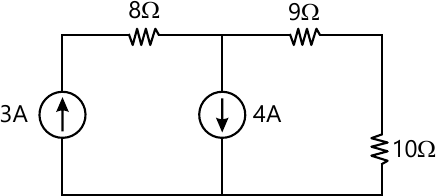3 M
1 (a) (ii) Determine current through 10Ω resistance :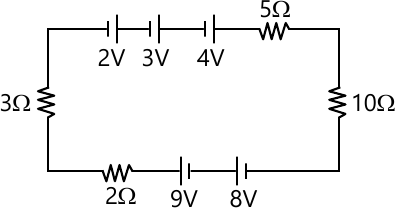2 M
1 (b) Find Laplace transform of signal :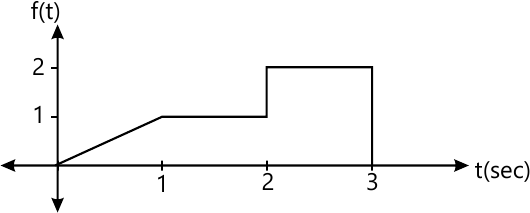5 M
1 (c) Find VC1 (f) when switch S is closed at t=0, with initial conditions :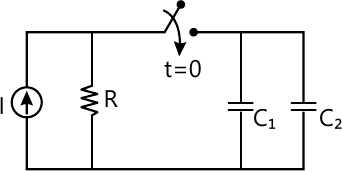5 M
1 (d) Determine the Z parameters of the network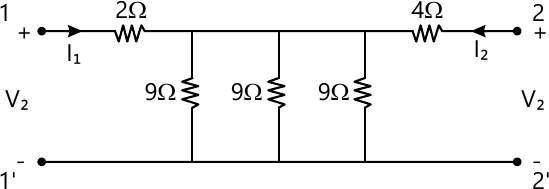5 M
1 (e) "Find the domain response using graphical & partial fraction method & prove that results are matching
$F \left(S \right)=\frac{3s}{\left(s+6 \right)\left(s+2 \right)}$ "
5 M

2 (a) (i) State and explain significance of initial & find value theorems. (Network Analysis)
5 M
2 (a) (ii) Derive the equation for Laplace transform of following functions :
(i) Unit Ramp function
Unit impulse function
5 M
2 (b) Determine RL for maximum power transfer & maximum power transferred PL
: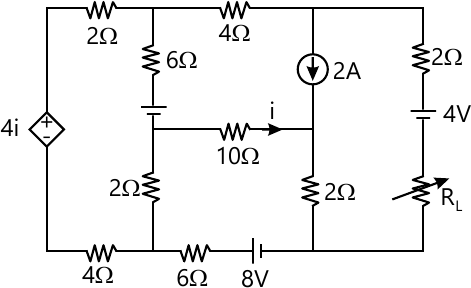10 M

3 (a) (i) In case of series R-L circuit excited by DC supply (V) derive equation for transient current IL with initial conditions.
5 M
3 (a) (ii) Define transmission line parameters in case of two port network. Also derive the condition of symmetry.
5 M
3 (b) "The driving point impedance of a one port network function is as follows. Obtain Foster 1 and Foster 2 form of equivalent circuit.
$Z\left(s \right)=\frac{6\left(s^2+4 \right)s}{\left(s^2+1 \right)\left(s^2+64 \right)}$"
10 M

4 (a) Explain the concept of poles & zeros. Using suitable example plot pole-zero plot & hence explain how to use such plot to get time domain response for network function.
10 M
4 (b) "Realise given YLC(S) into cauer 2 form
$Y\left(s \right)=\frac{s^4+6s^2+4}{2s^3+4s}$ "
10 M

5 (a) (i) "Check the following function for positive real function $(i) Z\left(s \right)=\frac{6\left(s^2+4 \right)s}{\left(s^2+1 \right)\left(s^2+64 \right)}$
$\left(ii \right) Z\left(s \right)=\frac{s\left(s^2+3 \right)}{\left(s^2+1 \right)}$ "
5 M
5 (a) (ii) "Check the following polynomials for Hurwitz
$\left(1 \right) P\left(s \right)=s^5+4s^4+3s^3+s^2+4s+1 \\ \left(2 \right) P\left(s \right)=s^4+4s^2+8$"
5 M
5 (b) (i) Obtain ABCD parameters for following two port networks. : (1)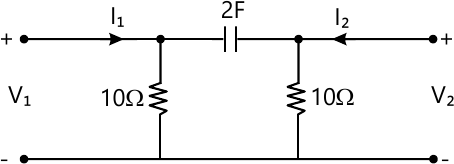5 M
5 (b) (ii)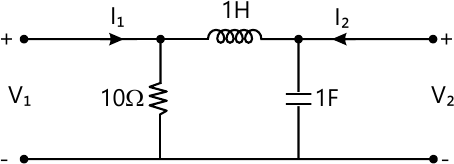5 M

6 (a) (i) Derive network equilibrium equation on loop current basis (KVL).
5 M
6 (a) (ii) For the network, get incidence matrix & tieset matrix :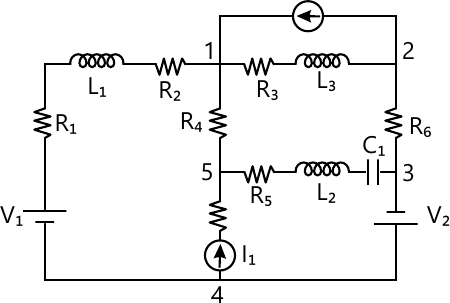5 M
6 (b) "For the network determine $i_1, i_2, \frac{di_1}{dt}, \frac{di_2}{dt}, \frac{d^2i_1}{dt^2}, \frac{d^2i_2}{dt^2}$
for t= 0+. The switch is closed at t=0. :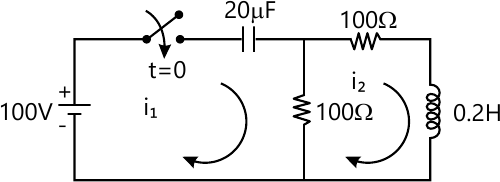"
10 M

7 (a) (i) Determine current through 10Ω resistance for the network :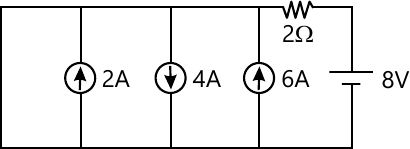5 M
7 (a) (ii) For the network, Switch S is closed at t=0 with initial conditions as shown. Determine VR (t)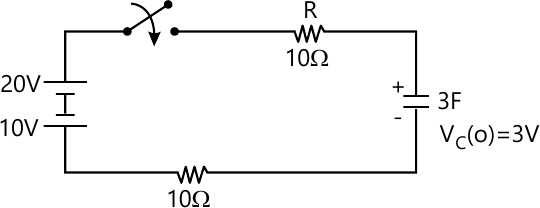5 M
7 (b) Using Superposition theorem, determine current through 10 Ω resistance. :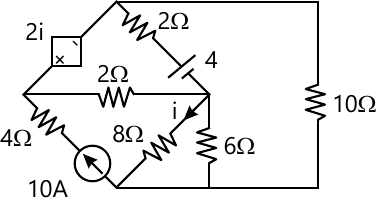10 M

More question papers from Circuits and Transmission Lines